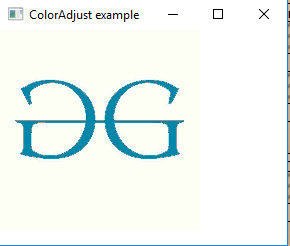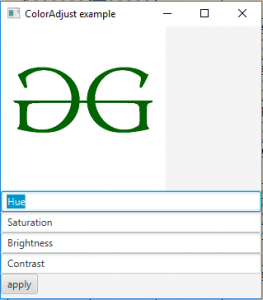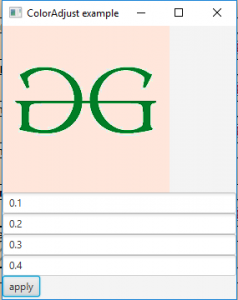ColorAdjust class is a part of JavaFX. ColorAdjust class allows per-pixel adjustments of hue, saturation, brightness, and contrast. ColorAdjust class inherits Effect class.

Constructors of the class:

2. ColorAdjust(double hue, double saturation, double brightness, double contrast): Creates a new Object of ColorAdjust class with specified values of hue, satuartion, brightness and contrast.

Commonly Used Methods:

Methods Explanation
getBrightness() Returns the value of brightness of ColorAdjust effect
setBrightness(double v) Sets the value of brightness of ColorAdjust effect
getHue() Returns the value of Hue of ColorAdjust effect
setHue(double v) Sets the value of Hue of ColorAdjust effect
getContrast() Returns the value of Contrast of ColorAdjust effect
setContrast(double v) Sets the value of Contrast of ColorAdjust effect
getSaturation() Returns the value of Saturation of ColorAdjust effect
setSaturation(double v) Sets the value of Saturation of ColorAdjust effect
getInput() Returns the value of property input
setInput(Effect v) Sets the value of property input

Below programs illustrate the use of ColorAdjust class:

1. Java Program to apply color Adjust effect to a image with specified hue, brightness, contrast and Saturation: In this program a FileInputStream is created and an image is taken as input from a file. Image named image is created using the input from file input stream. From the image a image view object is created and it is added to the VBox. The VBox is then added to the scene and the scene is added to the stage. A ColorAdjust effect is created and the values for hue, saturation, contrast and brightness are set using function setHue(), setBrightness(), setSaturation(), setContrast() and the effect is set to the image view using setEffect() function.

 `// Java Program to apply color Adjust effect ` `// to a image with specified hue, brightness,  ` `// contrast and Saturation ` `import` `javafx.application.Application; ` `import` `javafx.scene.Scene; ` `import` `javafx.scene.control.*; ` `import` `javafx.scene.layout.*; ` `import` `javafx.stage.Stage; ` `import` `javafx.scene.image.*; ` `import` `javafx.scene.effect.*; ` `import` `java.io.*; ` `import` `javafx.event.ActionEvent; ` `import` `javafx.event.EventHandler; ` `import` `javafx.scene.Group; ` ` `  `public` `class` `ColorAdjust_1 ``extends` `Application { ` ` `  `    ``// launch the application ` `    ``public` `void` `start(Stage stage) ``throws` `Exception ` `    ``{ ` ` `  `        ``// set title for the stage ` `        ``stage.setTitle(``"ColorAdjust example"``); ` ` `  `        ``// create a input stream ` `        ``FileInputStream input = ``new` `FileInputStream(``"f:\\gfg.png"``); ` ` `  `        ``// create a image ` `        ``Image image = ``new` `Image(input); ` ` `  `        ``// create a image View ` `        ``ImageView imageview = ``new` `ImageView(image); ` ` `  `        ``// create a ColorAdjust effect ` `        ``ColorAdjust color_adjust = ``new` `ColorAdjust(); ` ` `  `        ``// set hue, saturation, brightness, and contrast ` `        ``color_adjust.setHue(``0.4``); ` `        ``color_adjust.setBrightness(``0.6``); ` `        ``color_adjust.setContrast(``0.8``); ` `        ``color_adjust.setSaturation(``0.1``); ` ` `  `        ``// set effect ` `        ``imageview.setEffect(color_adjust); ` ` `  `        ``// create a VBox ` `        ``VBox vbox = ``new` `VBox(imageview); ` ` `  `        ``// create a scene ` `        ``Scene scene = ``new` `Scene(vbox, ``200``, ``200``); ` ` `  `        ``// set the scene ` `        ``stage.setScene(scene); ` ` `  `        ``stage.show(); ` `    ``} ` ` `  `    ``// Main Method ` `    ``public` `static` `void` `main(String args[]) ` `    ``{ ` ` `  `        ``// launch the application ` `        ``launch(args); ` `    ``} ` `} `

Input Image:Output:2. Java Program to apply color Adjust effect to an image with hue, brightness, contrast, and Saturation is taken as input from the user(using text fields): In this program a FileInputStream is created and an image is taken as input from a file. Image named image is created using the input from the file input stream. From the image, an image view object is created and it is added to the VBox. The VBox is then added to the scene and the scene is added to the stage. A ColorAdjust effect is created and the values for hue, saturation, contrast and brightness are set using function setHue(), setBrightness(), setSaturation(), setContrast() and the effect is set to the image view using setEffect() function. We will create four text fields(hue, saturation, contrast, and brightness) and a Button button. The user will give the necessary values of hue, saturation, contrast, and brightness and the values will be applied to the image when the button is pressed. An EventHandler is created to handle the button events.

 `// Java Program to apply color Adjust effect ` `// to a image with hue, brightness, contrast ` `// and Saturation taken as input from from user ` `import` `javafx.application.Application; ` `import` `javafx.scene.Scene; ` `import` `javafx.scene.control.*; ` `import` `javafx.scene.layout.*; ` `import` `javafx.stage.Stage; ` `import` `javafx.scene.image.*; ` `import` `javafx.scene.effect.*; ` `import` `java.io.*; ` `import` `javafx.event.ActionEvent; ` `import` `javafx.event.EventHandler; ` `import` `javafx.scene.Group; ` ` `  `public` `class` `ColorAdjust_2 ``extends` `Application { ` ` `  `// launch the application ` `public` `void` `start(Stage stage) ``throws` `Exception ` `{ ` ` `  `    ``// set title for the stage ` `    ``stage.setTitle(``"ColorAdjust example"``); ` ` `  `    ``// textfields ` `    ``TextField hue, saturation, brightness, contrast; ` ` `  `    ``// create the textFields ` `    ``hue = ``new` `TextField(``"Hue"``); ` `    ``saturation = ``new` `TextField(``"Saturation"``); ` `    ``brightness = ``new` `TextField(``"Brightness"``); ` `    ``contrast = ``new` `TextField(``"Contrast"``); ` ` `  `    ``// create a input stream ` `    ``FileInputStream input = ``new` `FileInputStream(``"f:\\gfg.png"``); ` ` `  `    ``// create a image ` `    ``Image image = ``new` `Image(input); ` ` `  `    ``// create a image View ` `    ``ImageView imageview = ``new` `ImageView(image); ` ` `  `    ``// create a ColorAdjust effect ` `    ``ColorAdjust color_adjust = ``new` `ColorAdjust(); ` ` `  `    ``// create a button ` `    ``Button button = ``new` `Button(``"apply"``); ` ` `  `    ``// action event ` `    ``EventHandler event = ``new` `EventHandler() { ` `        ``public` `void` `handle(ActionEvent e) ` `        ``{ ` `            ``// set the hue, brightness, contrast and saturation ` `            ``color_adjust.setHue(Double.parseDouble(hue.getText())); ` `             `  `            ``color_adjust.setBrightness(Double.parseDouble( ` `                                     ``brightness.getText())); ` `                                      `  `            ``color_adjust.setContrast(Double.parseDouble( ` `                                    ``contrast.getText())); ` `                                     `  `            ``color_adjust.setSaturation(Double.parseDouble( ` `                                     ``saturation.getText())); ` `        ``} ` `    ``}; ` ` `  `    ``// set on action of button ` `    ``button.setOnAction(event); ` ` `  `    ``// set effect ` `    ``imageview.setEffect(color_adjust); ` ` `  `    ``// create a VBox ` `    ``VBox vbox = ``new` `VBox(imageview, hue, saturation,  ` `                    ``brightness, contrast, button); ` ` `  `    ``// create a scene ` `    ``Scene scene = ``new` `Scene(vbox, ``200``, ``400``); ` ` `  `    ``// set the scene ` `    ``stage.setScene(scene); ` ` `  `    ``stage.show(); ` `} ` ` `  `// Main Method ` `public` `static` `void` `main(String args[]) ` `{ ` ` `  `    ``// launch the application ` `    ``launch(args); ` `} ` `} `

Input Image:Output:Note: The above programs might not run in an online IDE. Please use an offline compiler.

Attention reader! Don’t stop learning now. Get hold of all the important DSA concepts with the DSA Self Paced Course at a student-friendly price and become industry ready.

My Personal Notes arrow_drop_upCheck out this Author's contributed articles.

If you like GeeksforGeeks and would like to contribute, you can also write an article using contribute.geeksforgeeks.org or mail your article to contribute@geeksforgeeks.org. See your article appearing on the GeeksforGeeks main page and help other Geeks.

Please Improve this article if you find anything incorrect by clicking on the "Improve Article" button below.

Article Tags :
Practice Tags :

Be the First to upvote.

Please write to us at contribute@geeksforgeeks.org to report any issue with the above content.